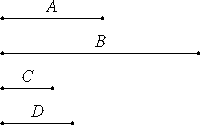# Proposition 16

If a square does not measure a square, then neither does the side measure the side; and, if the side does not measure the side, then neither does the square measure the square.

Let A and B be square numbers, and let C and D be their sides, and let A not measure B.

I say that neither does C measure D.VIII.14

If C measures D, A also measures B. But A does not measure B, therefore neither does C measure D.

Next, let C not measure D.

I say that neither does A measure B.

VIII.14

If A measures B, then C also measures D. But C does not measure D, therefore neither does A measure B.

Therefore, if a square does not measure a square, then neither does the side measure the side; and, if the side does not measure the side, then neither does the square measure the square.

Q.E.D.

## Guide

This is simply the contrapositive of VIII.14. It is unclear why papyrus was wasted to state and prove it.